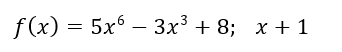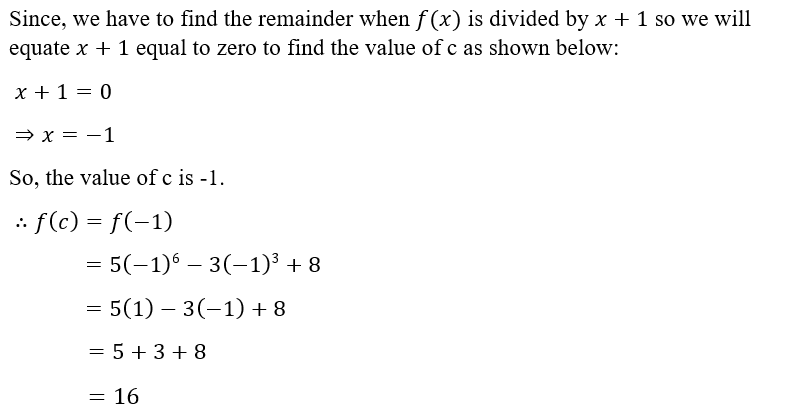# Use the Remainder Theorem to find the remainder when f(x) is divided by x-cf(x)= 5x6-3x3+8; x+1

Question
2 views

Use the Remainder Theorem to find the remainder when f(x) is divided by x-c

f(x)= 5x6-3x3+8; x+1

check_circle

Remainder Theorem:

The remainder theorem states that whenever polynomial function f(x) is divided by (x− c) the remainder is given by evaluating f(c).

Given:Solution:...

### Want to see the full answer?

See Solution

#### Want to see this answer and more?

Solutions are written by subject experts who are available 24/7. Questions are typically answered within 1 hour.*

See Solution
*Response times may vary by subject and question.
Tagged in